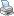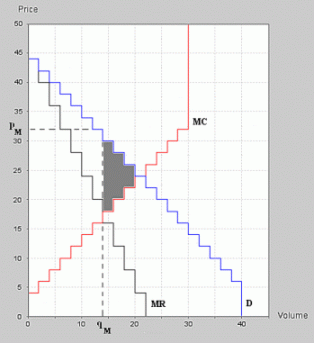Collapse Sidebar

 Students / SubjectsExperiments > MarketLink > Monopoly Market ExperimentsPrinter Friendly

Monopoly Market Experiments

The monopoly model predicts that a monopolist chooses the output qM that equates its marginal revenue and marginal cost, and selects the price pM so that it sells quantity qM. Figure 1 shows several important features from the monopoly model. The seller's marginal revenue, labelled MR, lies below the demand 'D'. The seller's marginal cost, labelled MC, intersects MR at qM = 14, and the monopolist charges the price pM = 32.Figure 1: Monopoly experiment.

The area above the price and below the demand is the consumers' surplus. In this example it is 84, which is substantially less than the consumers' surplus that would be obtained if the price were at the intersection of supply and demand, as is the case in the competitive market which has consumers' surplus 220. The monopolist's profit in this example is 308, which is much greater than the profit at the competitive price (also 220).

One of the arguments against monopoly is that there is a surplus loss due to the restricted output by the monopolist. The monopolist's output only 14. At the competitive price pc = 24, there are 20 units that trade with a surplus. The surplus on these last six units, shown as the shaded area in the figure, is called the monopoly deadweight loss.

One of the advantages of a monopoly experiment is that each of these predictions can be tested. The number of trades is observed, the average price can be computed, the surplus of the buyers can be calculated, and so can the seller's profit. MarketLink experiments that test the competitive equilibrium predictions have been prepared for both the double auction and the posted offer auction.

To add the configuration for a double auction monopoly market profile, click the button below.

To add the configuration for a posted offer monopoly market to your profile, click the button below.